Select Page

# CBSE Maths 12 Science Vector Algebra MCQ Answers in English

CBSE Maths 12 Science Vector Algebra MCQ Answers in English to enable students to get Answers in a narrative video format for the specific question.

Expert Teacher provides CBSE Maths 12 Science Vector Algebra MCQ Answers through Video Answers in English language. This video solution will be useful for students to understand how to write an answer in exam in order to score more marks. This teacher uses a narrative style for a question from Vector Algebra not only to explain the proper method of answering question, but deriving right answer too.

Please find the question below and view the Answer in a narrative video format.

Question:

## Similar Questions from CBSE, 12th Science, Maths, Vector Algebra

Question 1 :  If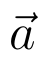and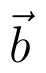denote the position vectors of points A and B respectively and C is a point on AB such that AC = 2 CB, then write the position vector of C.  (View Answer Video)

Question 2 : If the vertices A, B, C of a triangle ABC have position vectors (1, 2, 3), (-1, 0, 0), (0, 1, 2) respectively then find <ABC (<ABC is the angle between the vectors BA and BC). (View Answer Video)

Question 3 : Find |a| and |b|, if (a + b).(a - b) = 8 and |a| = 8|b|. (View Answer Video)

Question 4 : Find the scalar components of the vector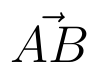with initial point A (2, 1) and terminal point B (-5, 7).  (View Answer Video)

Question 5 : Find the vector quantities :
(i)     Work                     (ii)      Force

### Integrals

Question 1 : Let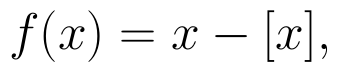for every real number, x, where [x] is the greatest integer less than or equal to x.
Evaluate :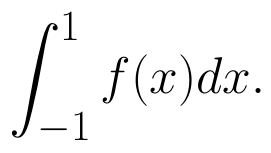(View Answer Video)

Question 2 : Evaluate :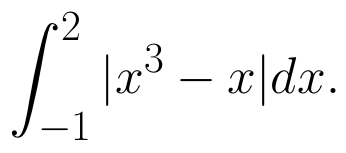(View Answer Video)

Question 3 : Find the integral of the function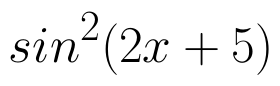. (View Answer Video)

Question 4 : Evaluate :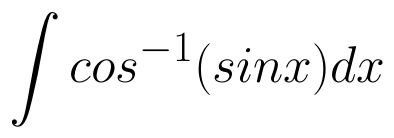. (View Answer Video)

Question 5 :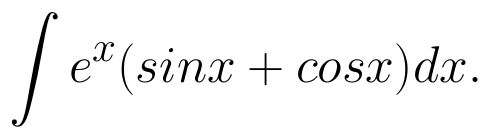(View Answer Video)

### Relations and Functions

Question 1 : If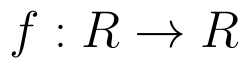is defined by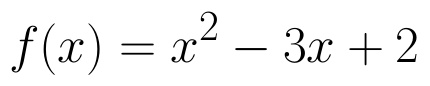, write f(f(x)). (View Answer Video)

Question 2 : Letdefined as f(x) = x be an identity function. Then, (View Answer Video)

Question 3 : Number of binary operations on the set { a, b} are: (View Answer Video)

Question 4 : The identity element for the binary operation * defined by a * b =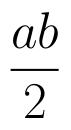, where a, b are the elements of a set of non-zero rational numbers, is, (View Answer Video)

Question 5 : Functions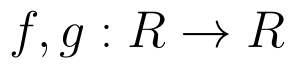are defined respectively, by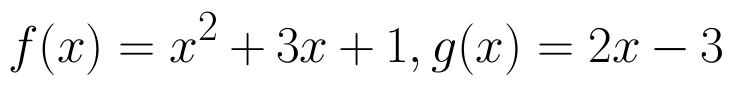, find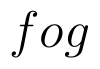. (View Answer Video)

### Application of Integrals

Question 1 : Find the area of the given curves and given lines: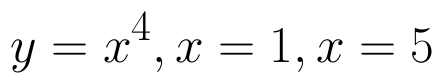and x-axis (View Answer Video)

Question 2 : Find the area of the region bounded by the curve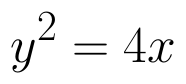and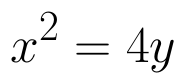. (View Answer Video)

Question 3 : Find the area of the region in the first quadrant enclosed by x-axis, line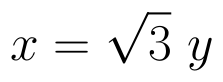and the circle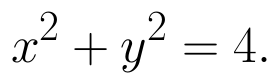(View Answer Video)

Question 4 : Using the method of integration, find the area of the triangular region whose vertices are (2, -2), (4, 3) and (1, 2). (View Answer Video)

Question 5 : Find the area of the region bounded by the parabola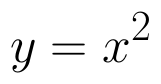and y = | x |. (View Answer Video)